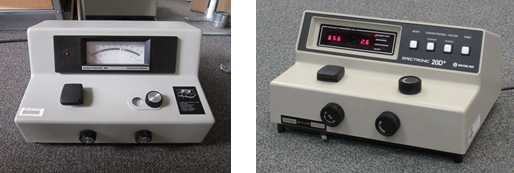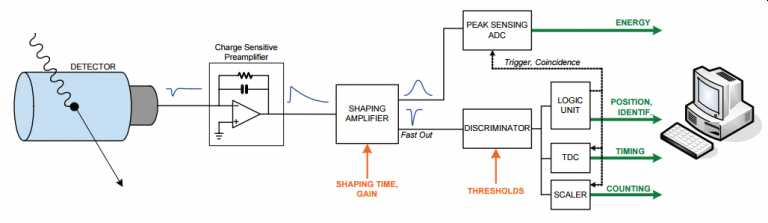# 7.6: Signal Processors and Readouts

$$\newcommand{\vecs}{\overset { \rightharpoonup} {\mathbf{#1}} }$$ $$\newcommand{\vecd}{\overset{-\!-\!\rightharpoonup}{\vphantom{a}\smash {#1}}}$$$$\newcommand{\id}{\mathrm{id}}$$ $$\newcommand{\Span}{\mathrm{span}}$$ $$\newcommand{\kernel}{\mathrm{null}\,}$$ $$\newcommand{\range}{\mathrm{range}\,}$$ $$\newcommand{\RealPart}{\mathrm{Re}}$$ $$\newcommand{\ImaginaryPart}{\mathrm{Im}}$$ $$\newcommand{\Argument}{\mathrm{Arg}}$$ $$\newcommand{\norm}{\| #1 \|}$$ $$\newcommand{\inner}{\langle #1, #2 \rangle}$$ $$\newcommand{\Span}{\mathrm{span}}$$ $$\newcommand{\id}{\mathrm{id}}$$ $$\newcommand{\Span}{\mathrm{span}}$$ $$\newcommand{\kernel}{\mathrm{null}\,}$$ $$\newcommand{\range}{\mathrm{range}\,}$$ $$\newcommand{\RealPart}{\mathrm{Re}}$$ $$\newcommand{\ImaginaryPart}{\mathrm{Im}}$$ $$\newcommand{\Argument}{\mathrm{Arg}}$$ $$\newcommand{\norm}{\| #1 \|}$$ $$\newcommand{\inner}{\langle #1, #2 \rangle}$$ $$\newcommand{\Span}{\mathrm{span}}$$$$\newcommand{\AA}{\unicode[.8,0]{x212B}}$$

While older instruments such as the Milton Roy Spectronic 20 pictured on the left in Figure 7.6.1 was a purely analog instrument all laboratory grade instruments are digital.  In an analog instrument such as the Spectronic 20 the current from the vacuum phototube is amplified and used to drive the front panel meter.   Today if you purchased the equivalent instrument pictured on the right in figure 7.6.1, the Spectronic 20 Model D, you will note that the meter has been replaced by a digital display.Figure$$\PageIndex {1}$$: Two Spectronic 20 or Spec 20 instruments for absorption spectroscopy in the visible region of the spectrum.  teh instrumetn on the left is an older analog spectrophotometer and the right on the right is a newer spectrophotometer

In both cases, the small current produced by the vacuum phototube is amplified close to the source using a circuit like that shown in Figure 7.6.2, in this case for a photodiode.  The difference is in an analog instrument the voltage coming from the current to voltage converter is further amplifier and used to drive the meter in an analog instrument or directly converted to a number by an analog to digital converter.Figure$$\PageIndex {2}$$: An illustration of a the circuit used for current to voltage conversion and signal amplification.

Analog to Digital (A/D) converters are common electronic components in all modern instrumentation.  16 to 20 bit A/Ds are readily available at low cost and introduce a digitization error of 1 part in 216 (better than 1 part in 65,000) for a 16-bit A/D.

For low light level experiments using photomultiplier tubes, such as in emission spectroscopy, a similar signal train of amplification and digitization is possible.  An alternative signal processing method is photon counting.  As shown in Figure  7.6.3, the signal from the photomultiplier tube is amplified and converted to a voltage pulse by a circuit similar to that shown in Figure 7.6.2. In photon counting, the voltage pulse is shaped into a standard form, compared to a voltage threshold in the discriminator and if larger than the set voltage value in the discriminator counted.   The number of counts for a specified period of time will be proportional to the intensity of light striking the detector.Figure$$\PageIndex {3}$$: An illustration of a data processing scheme for photon counting.  Not discussed in this section is the peak height analysis involving the peak sensing ADC that is useful in X-ray fluorescence spectroscopy for determining the energy of the photon. This image was copied from the physicsopenlab.org

7.6: Signal Processors and Readouts is shared under a not declared license and was authored, remixed, and/or curated by LibreTexts.